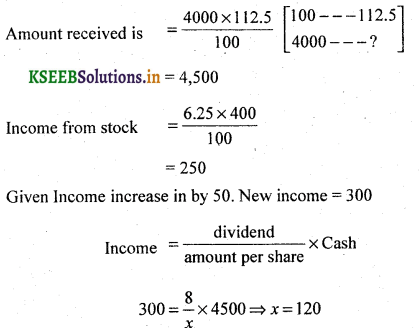# 2nd PUC Basic Maths Question Bank Chapter 9 Stocks and Shares

Students can Download Basic Maths Question Bank Chapter 9 Stocks and Shares Questions and Answers, Notes Pdf, 2nd PUC Basic Maths Question Bank with Answers helps you to revise the complete Karnataka State Board Syllabus and to clear all their doubts, score well in final exams.

## Karnataka 2nd PUC Basic Maths Question Bank Chapter 9 Stocks and Shares

### 2nd PUC Basic Maths Stocks and Shares One Mark Questions and Answers

Question 1.
Define Yield:
Yield is nominal interest divided by Amount invested.

Question 2.
What is the rate of interest obtained by investing in 9% stock at 180.
$$\text { Yield }=\frac{\text { Interest }}{\text { Amount }}=\frac{9}{180} \times 100=5 \%$$Question 3.
What income can be obtained from Rs. 3600 in 5% stock.
For 100 Rs. income is 5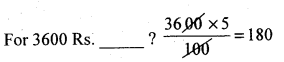∴ Income = 180Rs.

Question 4.
How much stock can be purchased by investing. Rs. 9600 in 3.5% stock at 120. Here if M.V. is 120 then face value =100
Here M.V. is 9600 then face value = 100
If M.V IS 9600 then face value∴ Amount of stock = 8000 Rs

Question 5.
What is the rate of interest by investing in 14.5% p.a. at 81?
Interest $$=\frac{14.5}{81} \times 100=17.9 \% \approx 18 \%$$

Question 6.
Calculate the yield on Rs. 1000 invested in 15% at Rs. 75.
$$Yield =\frac{\text { Interest }}{\text { Amount inversted per share }}=\frac{15}{75}=0.20$$Question 7.
Find the yield by Rs. 1,140 on 15% Stock quoted at Rs. 95.
$$Yield =\frac{\text { dividend }}{\text { amount invested per share }}=\frac{15}{95}=0.1578$$

Question 8.
What is the yield on Rs.1000 invested in 14% at 85?
Yield =$$\frac{\text { dividend }}{\text { amount invested / share }}=\frac{14}{85}=0.1647$$

Question 9.
10% Stock is Quoted at Rs. 120. Find the rate of Interest.
Interest = $$\frac{10}{120}$$x 100 = 8 .33%

### 2nd PUC Basic Maths Stocks and Shares Three or four Marks Questions and Answers

Question 10.
Which is better investment (i) 8% Stock at 110 or 9% stock at 98
Here we need to calculate the yield and see
Which stock gives higher returns.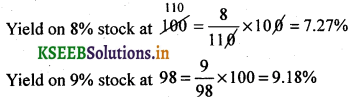∴ 9% stock at 98 is better.

Question 11.
$$6 \frac{1}{2} \%$$ Stock at 84 and 84% Stock at 102.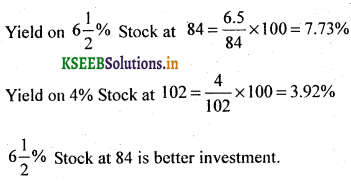Question 12.
What is the market value of 6% stock if it earns an interest of 4.5% after deducting the income tax of 4%.
Let the income = Rs. 6
4% tax deduction $$=\frac{4}{100} \times 6=0.24$$
∴ Net in come = 6 – 0.24 = 5.76
Given Yield after tax deduction = 4.5%∴ Market value of Rs. 100 Stock is Rs. 128.

Question 13.
If a person wishes to obtain 18% yield fram his investment, at what price should he buy 13.5% Stock.Question 14.
How much must be invested in 14.25% stock at 98 to produce the same income as could be obtained by investing Rs. 9,975 in 15% stock at 105?
Let the cash invested be x
Income from 14.25% stock at 98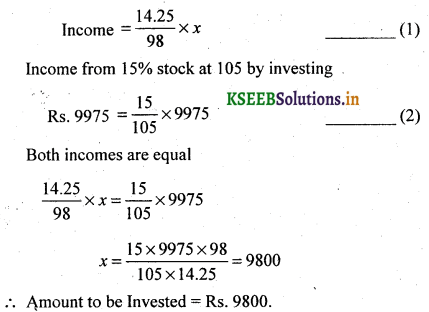Question 15.
A invests a sum of money in 5.5% stock at 90and B, equal sum in the 3.5%. If A’s income is 10% more than B’s. Find the price of the 3.5% stock.
Let A’s income $$=\frac{5.5}{90} \times x$$
Where x is the investment of A
Let B’s income $$=\frac{3.5}{y} \times x$$
Where y = is the price of 3.5% stock
Given A’s income is 10% more than B’s
We get A’s income : B’s income =110 : 100Question 16.
How much money has to be invested in 11.5% stock at 73 (Including brokerage) to obtain an income of Rs. 150 after a tax deduction at source of 20%.
Given net income =150 and tax deduction at 20%
Net income = 80 If gross income =100Question 17.
A person invests Rs. 15,000 cash partly in 3% stock at 75 and partly in 6% stock at 125 in such a way to get a return of 4.5% for his money. How much does he invest in each?
Let Rs. x be investment in 3% stock
and Rs. (15,000 – x) will be invested in 6% stock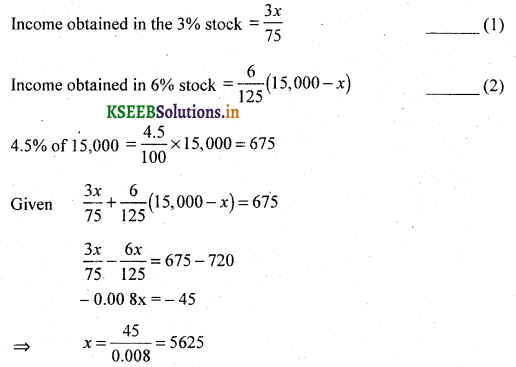Amount invested in 3% stock is Rs. 5625
& Amount invested in 6% stock is Rs. 15,000 – 5625 = Rs. 9375.Question 18.
Ravi sold Rs. 2,250 stock at 75 and bought stock at 88.f 9 with the proceeds. How much stock does he buy if the brokerage is 2% for selling and 1.5% for buying?
Since the brokerage for selling is 2%
Cost of 1 share is 75 – 2 = 73
Amount received by selling stock worth Rs. 2250 = ?
for 100 —- 73 M.V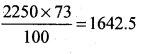Cost of 1 Share is 88.50 + 1.50 = 90  for 100 —— 90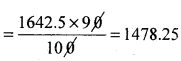Question 19.
Mr. Gopal invested Rs. 2,400 partly in 10% stock at 120 and partly in 12% stock at 96 find his investment in each. If the income derived from both the investments be Rs. 275.
Let the amount invested in 10%
stocked the remaining amount (2400 – x) will be invested in 12% stock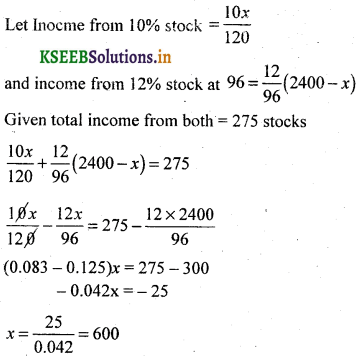∴ Amount invested in 10% stock is Rs. 600 and 12% stock is Rs. 1,800.

Question 20.
Kiran sold Rs. 800 stock at Rs. 91 and purchased stock at Rs. 94.50 with the proceeds. How much does he buy if the brokerage is 0.25%.
Cost of one share is 91 – 0.25 = 90.75
100 stock – Amount is 90.75
800 stock – ?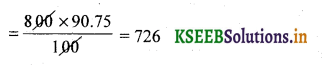Amount received by selling 800 stock = Rs. 726
Again he purchased stock for 94.50
Cost of one share = 94.50 + 0.25 = 94.75
For 100 ____ 94.75
? ____  726
∴ Kiran buys the stock worth $$=\frac{726 \times 100}{94.75}=766.22$$

Question 21.
A person invested 42,000 partly in 5% stock at 125 and the remaining in 7.5% stock at 75. If income derived from the two stocks is the same. Find the respective investments in each stock also find the total income.
Let Rs. x be invested in 5% stock
and (42,000 -x) be invested in 7.5% stock
Income obtained from 5% stock at $$125=\frac{5 x}{125}$$
Income obtained from 7.5 stock at $$75=\frac{7.5}{75}(42,000-x)$$
Given both Incomes are equals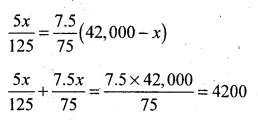0.04 x + 0.1 x = 4200
0.14 x = 4200
x = 30,000
∴ Rs. 30,000 in 5% stock and 12,000 in 7.5% stock
Total income 1200 + 1200 = 2400.Question 22.
Arun invests Rs. 15,000 partly in 3% stock at 75 and partly in 6% stock at 125. If the total interest income from both is Rs. 675. Find his investment in the two types of stock.
Let x be the investment in 1 st stock
and 15,000 -x be the investment in 2nd stock
Income in 1st stock = $$\frac{3}{75} \times x$$
Income in 2nd stock = $$\frac{6}{125} \times(15,000-x)$$
Given sum of both incomes = 675∴ Amount invested in 1st stock = 5625
and Amount invested 2nd stock = 15,000 – 5625 = Rs. 9375.

Question 23.
An investor sold Rs. 500 stock at Rs. 89.50 and buys stock at Rs. 92.50 with the proceeds. How much stock he buys the brokerage being 0.125%.
cost of 1 share = 89.50 – 0.125
after selling = 89.375
Amount received by selling Rs. 500 stockQuestion 24.
Rahul invests Rs. 1,74,000 partly in 12% stock and partly in 15% stock at 86. If the total income from both is 24,000. Find the amount invested by Rahul in 12% stock.